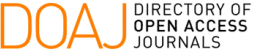### Construction of Complex Lattice Codes via Cyclotomic Fields

E. D. Carvalho, A. A. Andrade, T. Shah, C. C. Trinca

#### Abstract

Through algebraic number theory and Construction $A$ we extend an algebraic procedure which generates complex lattice codes from the polynomial ring \mathbb{F}_{2}[x]/(x^{n}-1), where \mathbb{F}_{2}=\{0,1\}, by using ideals from the generalized polynomial ring \frac{\mathbb{F}_{2}[x,\frac{1}{2}\mathbb{Z}_{0}]}{((x^{\frac{1}{2}})^{n}-1)} through the ring of integers $\mathcal{O}_{\mathbb{L}}$ of the cyclotomic field \mathbb{L}=\mathbb{Q}(\zeta_{2^{s}}), where \zeta_{2^{s}} is a 2^{s}-th root of the unit, with s>2.

#### Full Text:

PDF

DOI: https://doi.org/10.5540/tcam.2022.023.01.00033

### Refbacks

• There are currently no refbacks.

Trends in Computational and Applied Mathematics

A publication of the Brazilian Society of Applied and Computational Mathematics (SBMAC)

Indexed in: